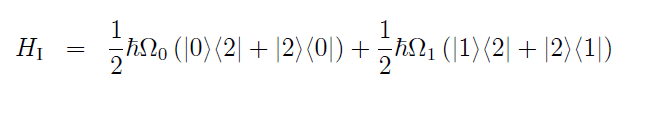# Eigenvalues of Hamiltonian

## Homework Statement

Find the eigenvalues of the followingand the eigenvelctor which corresponds to the smallest eigenvalue

## Homework Equations

I know how to find the eigenvalues and eigenvectors of a 2x2 matric but this one I'm not so sure so any help would be appreciated

## Answers and Replies

Since the hamiltonian consists of the states |0> , |1> and |2> , these will be a basis for it's non-zero eigenvalue eigenstates, because, if you acted with the hamiltonian to every other state |n>, you will get zero (assuming that |n>'s are a complete set of orthonormal states). So, try to act with this hamiltonian to a linear combination of the states |0> , |1> and |2> and then try to find the values of the coefficients of that superposition, that make this supreposition an eigenstate. This procedure will lead you to a homogeneus 3x3 system w.r.t. the coefficients, which, in order to have non-zero solutions, has to have a singular matrix (zero determinant). This condition will give you the eigenvalues and then, solvning the system for each eigenvalue, you will find the eigenstates.

If you have any problem with the above prescription, let me know so I can give you extra hints...

Last edited:
With the 2x2 matrixies I am used to making det(A-λI)=0 to find the eigenvalues and then solving the Aγ=λγ equation to get the eigenvectors, can I not sue a similar method here?

This procedure will lead you to a homogeneus 3x3 system w.r.t. the coefficients, which, in order to have non-zero solutions, has to have a singular matrix (zero determinant)

I just added this...

I don't massively understand, sorry! In lectures we have been told that there is a zero valued eigenvalue and I also know the corresponding eigenvalue. However I'm still unsure and don't really follow your method?

Start with this:
…a linear combination of the states |0> , |1> and |2> …
Consider this linear combination:

$$\left| {{\psi }_{i}} \right\rangle ={{c}_{i0}}\left| 0 \right\rangle +{{c}_{i1}}\left| 1 \right\rangle +{{c}_{i2}}\left| 2 \right\rangle$$

… then try to find the values of the coefficients of that superposition, that make this supreposition an eigenstate …

These superpositions will be eigenstates if:

$${{H}_{I}}\left| {{\psi }_{i}} \right\rangle ={{E}_{i}}\left| {{\psi }_{i}} \right\rangle$$

and this requirement will lead you to (I have set Ei = $\hbar$ωi/2):

$$\left[ {{\Omega }_{0}}{{c}_{i2}}\left| 0 \right\rangle +\left( {{\Omega }_{0}}{{c}_{i0}}+{{\Omega }_{1}}{{c}_{i1}} \right)\left| 2 \right\rangle +{{\Omega }_{1}}{{c}_{i2}}\left| 1 \right\rangle \right]={{\omega }_{i}}\left( {{c}_{i0}}\left| 0 \right\rangle +{{c}_{i1}}\left| 1 \right\rangle +{{c}_{i2}}\left| 2 \right\rangle \right)$$

Since |0> , |1> and |2> are linearly independent, the above relation will hold iff:

\begin{align} & {{\Omega }_{0}}{{c}_{i2}}={{\omega }_{i}}{{c}_{i0}} \\ & {{\Omega }_{0}}{{c}_{i0}}+{{\Omega }_{1}}{{c}_{i1}}={{\omega }_{i}}{{c}_{i1}} \\ & {{\Omega }_{1}}{{c}_{i2}}={{\omega }_{i}}{{c}_{i2}} \\ \end{align}

This is the 3x3 system that you have to solve…

Cheers, I'll give it a go, thanks for your help

I can't even solve that 3x3 system, any chance of another pointer?

\begin{align} & {{\Omega }_{0}}{{c}_{i2}}={{\omega }_{i}}{{c}_{i0}} \\ & {{\Omega }_{0}}{{c}_{i0}}+{{\Omega }_{1}}{{c}_{i1}}={{\omega }_{i}}{{c}_{i1}} \\ & {{\Omega }_{1}}{{c}_{i2}}={{\omega }_{i}}{{c}_{i2}} \\ \end{align}

This is the 3x3 system that you have to solve…

I made a mistake... This is the system:

\begin{align} & {{\Omega }_{0}}{{c}_{i2}}={{\omega }_{i}}{{c}_{i0}} \\ & {{\Omega }_{1}}{{c}_{i2}}={{\omega }_{i}}{{c}_{i1}} \\ & {{\Omega }_{0}}{{c}_{i0}}+{{\Omega }_{1}}{{c}_{i1}}={{\omega }_{i}}{{c}_{i2}} \\ \end{align}

Now, setting:

$A=\left( \begin{matrix} 0 & 0 & {{\Omega }_{0}} \\ 0 & 0 & {{\Omega }_{1}} \\ {{\Omega }_{0}} & {{\Omega }_{1}} & 0 \\ \end{matrix} \right)$ and ${{X}_{i}}=\left( \begin{matrix} {{c}_{i0}} \\ {{c}_{i1}} \\ {{c}_{i2}} \\ \end{matrix} \right)$

the system can be written in the matrix form:

$$A{{X}_{i}}={{\omega }_{i}}{{X}_{i}}$$

This is an eigenvalues equation. Can you solve it?